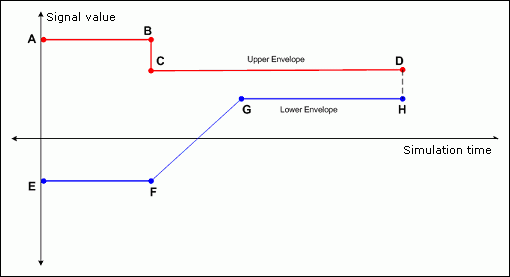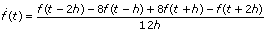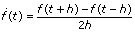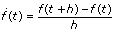# Defining Inequality Constraints (Control Design and Simulation Module)

LabVIEW 2018 Control Design and Simulation Module Help

Edition Date: March 2018

Part Number: 371894J-01

»View Product InfoDownload Help (Windows Only)

Inequality constraints represent trade-offs implicit in the problem specification. For example, you might be able to remove errors in a control loop by applying a very large control action. However, the necessary control action might be impossible to achieve in the real world. If you specify constraints on the control action before executing the Sequential Quadratic Programming (SQP) algorithm, you can eliminate optimal values that require an unfeasible control action.Note  Constraints add a great deal of complexity to the optimization problem. If possible, minimize the number of constraints before executing the SQP algorithm. One strategy to minimize the number of constraints involves first finding optimal values with no constraints, then gradually adding constraints and determining the least amount of constraints required for the dynamic system.

Because the optimization problem is based on a finite-horizon time-domain simulation, you specify the inequality constraints as envelopes that bound the time response of the control action and the output. You also can place inequality constraint envelopes on the rate of change of the control action and the rate of change of the output.

These envelopes are piecewise linear curves that specify the upper and lower limits on a signal during the simulation. The SIM Optimal Design VI then calculates H(p) as the minimum and maximum distance of the time series points from these envelopes.

Use the Inequality Constraints parameter of the SIM Optimal Design VI to define these envelopes. This parameter specifies the upper and lower constraint envelopes on the following four areas of the dynamic system: the control action, the output, the rate of change of the control action, and the rate of change of the output.Note  You can use the Graphically Specify Inequality Constraints VI, located in the labview\examples\Control and Simulation\Simulation\Optimal Control Design\Graphically Specify Inequality Constraints directory, to draw the upper and lower envelopes. This VI returns a set of points you then can wire to the Inequality Constraints parameter.

For example, consider an output yi(t) constrained by envelopes, as shown in the following illustration.A, B, C, and D are points that define the upper envelope UEi(t), and E, F, G, and H are points that define the lower envelope LEi(t). The SIM Optimal Design VI then constrains yi(t) to the following relationship:

LEi(t) < yi(t) < UEi(t)

This VI encodes this constraint by computing the clearance between the output and each envelope. The upper clearance UCi is defined as max (UEi(t) –  yi(t)). The lower clearance LCi is defined as max (yi(t) –  LEi(t)). These clearances clarify that the constraints must be positive, as the following relationships show:

–ε < UCi < ∞

–ε < LCi < ∞

where ε = 1E–21.

You also can place constraints on the rate of change of control actions and outputs. If at least five points are available, this VI computes these rates of change using the following equation:where t is the simulation time, h is the space between time steps, and f(t) is an output or control action signal.

At boundaries, or if fewer than five points are available, this VI uses the following equations instead:orYou can use a VI template to specify custom calculations for implementing the inequality constraints. To load this template, in the New dialog box, select VI»From Template»Simulation»Optimal Design»Compute Inequality Constraints from the Create New tree. To see an example of how to define and manipulate these parameters, open the SIMopt Compute Inequality Constraints (Default) VI, located in the labview\vi.lib\Simulation\Optimization Based Design\Constraints directory. This VI implements the inequality constraints the previous equations specified.

After you save the custom inequality constraint calculations as a VI, you must specify the location of the custom function in the Problem Specification parameter of the SIM Optimal Design VI. Select User defined for the Inequality constraints type parameter and specify the path to the VI in the File path user defined inequality constraints path control.